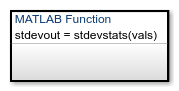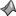## 通过定义 MATLAB 函数重用 MATLAB 代码

Stateflow® 图中的 MATLAB® 函数是一个图形元素，您可以用它来编写易于通过调用内置 MATLAB 函数来实现的算法。典型应用包括：

• 面向矩阵的计算

• 数据分析和可视化

MATLAB 函数可以驻留在 Stateflow 图、状态或子图的任意位置。函数的位置确定可以调用函数的状态和转移的集合。

• 如果想在一个状态（或子图）及其子状态内调用函数，请将您的 MATLAB 函数置于该状态或子图中。该函数将覆盖该状态或子图的父级和前代中同名的任何其他函数。

• 如果想要在图的任意位置调用函数，需将 MATLAB 函数置于与图同一层次的位置。

• 如果要从模型中的任何图调用该函数，请将您的 MATLAB 函数放在图级别，并启用图级别函数的导出。有关详细信息，请参阅Export Stateflow Functions for Reuse

MATLAB 函数可以访问在 Stateflow 层次结构中位于其上方的图和状态数据。```function stdevout = stdevstats(vals) %#codegen % Calculates the standard deviation for vals len = length(vals); stdevout = sqrt(sum(((vals-avg(vals,len)).^2))/len); function mean = avg(array,size) mean = sum(array)/size;```

### 在图中定义 MATLAB 函数

1. 在对象选项板中，点击 MATLAB 函数图标2. 在图画布上，点击新 MATLAB 函数的位置。

3. 输入函数的签名标签。

函数的签名标签指定函数的名称及其参数和返回值的形式名称。签名标签采用以下语法：

``[return_val1,return_val2,...] = function_name(arg1,arg2,...)``
您可以指定多个返回值和多个输入参数。每个返回值和输入参数可以是一个标量、向量或值的矩阵。对于只有一个返回值的函数，忽略签名标签中的方括号。

您可以对参数和返回值使用相同的变量名称。当您对输入和输出使用相同的变量时，您会创建原位数据。例如，使用以下签名标签的函数将变量 `y1``y2` 同时用作输入和输出：

`[y1,y2,y3] = f(y1,u,y2)`
如果将此函数导出为 C 代码，生成的代码会将 `y1``y2` 视为传引用的原位参数。使用原位数据可减少生成代码复制中间数据的次数，从而提高代码的效率。

符号窗格和模型资源管理器中，函数签名中的参数和返回值显示为属于您的函数的数据项。参数的作用域是“`输入`”。返回值的作用域是“`输出`”。

4. 为每个参数和返回值指定数据属性，如 设置数据属性 中所述。当参数和返回值同名时，只能编辑参数的属性。返回值的属性为只读。

5. 要对该函数进行编程，请双击函数框打开 MATLAB Function 模块编辑器。

6. 在 MATLAB Function 模块编辑器中，输入实现您的函数的 MATLAB 代码。有关详细信息，请参阅Program a MATLAB Function in a Chart

7. 创建您的函数所需的其他数据项。有关详细信息，请参阅通过模型资源管理器添加数据

您的函数可以访问它自己的数据或属于父状态或图的数据。函数中的数据项可以有以下作用域之一：

• `Constant` - 常量数据在所有函数调用中都保持其初始值。

• `Parameter` - 参数数据在所有函数调用中都保持其初始值。

在 MATLAB 函数中，不需要显式创建局部或临时函数数据。您可以使用未定义的变量来存储仅在函数调用的其余时间段可访问的值。要存储在各次函数调用之间保持不变的值，请在图级别使用局部数据或使用关键字 `persistent`

### 在状态和转移中调用 MATLAB 函数

``[return_val1,return_val2,...] = function_name(arg1,arg2,...)``

### 指定 MATLAB 函数的属性

1. 建模选项卡的设计数据下，选择

2. 在 Stateflow 编辑器中，选择 MATLAB 函数。

3. 属性检查器中，编辑转移属性。

1. 建模选项卡的设计数据下，选择

2. 模型层次结构窗格中，选择 MATLAB 函数。

3. 对话框窗格中，编辑 MATLAB 函数属性。

1. 在 Stateflow 编辑器中，右键点击 MATLAB 函数。

2. 选择属性

3. 在属性对话框中，编辑 MATLAB 函数属性。

#### 函数内联选项

• `自动`” - 确定是否根据内部计算内联函数。

• `内联`” - 如果不将函数导出到其他图并且它不是递归的一部分，则内联函数。（如果函数直接调用自身或通过另一个函数调用间接调用自身，则存在递归。）

• `函数`” - 不内联函数。

#### MATLAB Function `fimath`

• 与 MATLAB 相同 - 该函数使用与当前全局 `fimath` 相同的 `fimath` 属性。编辑框变暗，并以只读形式显示当前全局 `fimath`。有关全局 `fimath``fimath` 对象的详细信息，请参阅 Fixed-Point Designer™ 文档。

• 指定其他 - 通过以下方法之一指定您自己的 `fimath` 对象：

• 在编辑框内构造 `fimath` 对象。

• 在 MATLAB 或模型工作区中构造 `fimath` 对象，并在编辑框中输入其变量名称。

MATLAB 函数的描述。

#### 文档链接

MATLAB 函数的在线文档的链接。您可以输入 Web URL 地址或者将文档显示为 HTML 文件或 MATLAB 命令行窗口中的文本的 MATLAB 命令。点击文档链接超链接时，Stateflow 会计算该链接并显示该文档。